$B HMM Using Rhythm Vectors $B>e$X(B: Automatic Rhythm Transcription from $BLa$k(B: Introduction # Rhythm and Tempo The observed duration (IOI)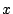[sec] of note in the performed MIDI data is related both to intended note value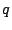[beats] in the score and to tempo variable[sec/beat] (average time per beat) by: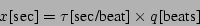(1) Rhythm recognition can be defined as a decomposition of IOIs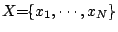into tempo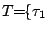,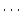,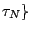and rhythm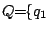,,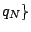. This is a kind of ill-posed problem since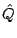and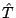are not determined uniquely. In principle, any rhythm can be expressed in various ways, e.g. twice note values and half tempo gives the same note duration in Eq. 1. Furthermore, fluctuation of tempo and rhythm can not be completely separated. Decomposition is possible only in a probabilistic sense assuming thatis constant or slowly changing (at least within phrases), and that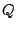often fit common rhythm patterns. Human often can recognize rhythm from the musical performance because they have a priori knowledge of rhythm, e.g., what type of rhythm patterns are likely to appear. In our approach, most likely rhythm patterns'' for the given MIDI data are estimated by search in the proposed probabilistic models, whose parameters are optimized by stochastic training with existing scores and performances. Our goal is to separate rhythm and tempo by iterating the estimation of the two. First, we estimate rhythm from the IOIs of the given MIDI using tempo-invariant feature parameters. Then, using the estimated rhythm and the given IOIs, the tempo is estimated. Rhythm and tempo are alternately re-estimated using the estimated counterpart. In the next sections, we discuss first two steps.$B HMM Using Rhythm Vectors $B>e$X(B: Automatic Rhythm Transcription from $BLa$k(B: Introduction
$BJ?@.(B16$BG/(B3$B7n(B25$BF|(B